# Switch X, Y axis of Inversed formula without chaning the inversed formula plot

#### Kylian187

##### New member
Hi,

I had some problems that I couldn't figure out by myself. I got the formula (100/(1023)0.67) * X0.67
This gives a slighty curved line with Y axis 100 and X axis 1023.

When I want to inverse this formula I get: Sqrt of 0.67 (x/(100/(1023)0.67)) (see picture)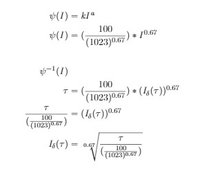But what happens is that the inverse of this formula got an Y of 1023 and X of 100.

My qeustion is there a way that this formula can be written so both plots have a Y of 100 and a X of 1023?

Kylian

#### Mr. Bland

##### Junior Member
An inverse function is one that attempts to "undo" a function--to reverse the process beginning with the output and determine what the input must have been: $$\displaystyle f^{-1}(f(x)) = x$$ (not every function has a 1:1 mapping, but this is the general idea). If you have a graph in the form of $$\displaystyle y = f(x)$$, you find the inverse of the function by solving for $$\displaystyle x$$ as a function of $$\displaystyle y$$, as in $$\displaystyle x = f^{-1}(y)$$.

That being said, if $$\displaystyle 100 = f(1023)$$, isn't it to be expected that $$\displaystyle 1023 = f^{-1}(100)$$?

If you use the inverse function as a function of $$\displaystyle x$$ unto itself such that $$\displaystyle y = f^{-1}(x)$$, you may find that the graph appears to exchange axes, reflecting over a 45-degree line from the origin.

•topsquark

#### Dr.Peterson

##### Elite Member
But there are no x and y in your work. What do you mean by them? Evidently in the original plot you are taking x to be I, and y to be $$\displaystyle \tau = \psi(I)$$, but when you plot the inverse you are swapping their roles. Of course you will not get the same x and y! They mean different things.

What's happening here is that in inverting the formula (that is, solving for I) when the variables have specific meanings, you have to keep the same variables (rather than swapping them as you do when you are working with a function of x, where x just means "the input of the function I am graphing").

So when you graph your inverse function, the labels on the axes will be swapped, and everything ought to make sense.

Note that you can't talk here about $$\displaystyle \psi^{-1}(I)$$. If you start with $$\displaystyle \tau = \psi(I)$$, then the inverse is $$\displaystyle I = \psi^{-1}(\tau)$$, not $$\displaystyle \psi^{-1}(I)$$. Luckily you didn't really do what you wrote.

•topsquark

#### Kylian187

##### New member
My bad let me explain what my thought sequence was. Hard time to explain what I ment.

It's about Steven powerlaw, it generates a graph on percieved items like light. Do undo these percieved items we use the inversion to counter the original graph so if we would combine them you would end up with X = Y.

For an example: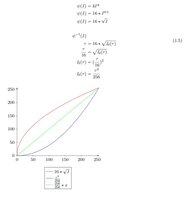This is what I would like to achieve exept this uses a ratio for X and Y as 256:256 so.
What I try is to get the same graph except with ratio of 1023:100.

Thanks for all the help

#### Mr. Bland

##### Junior Member
You want to scale the output, then? Right now it fits within the region of (0, 0)-(256, 256), but you would like it to instead fit into (0, 0)-(1023, 100)?

If the following is in the 256, 256 range...

$$\displaystyle y = f(x)$$​

... then the following is in the 1023, 100 range:

$$\displaystyle y = \frac{100}{256}f\left(\frac{1023}{256}x\right)$$​

#### Dr.Peterson

##### Elite Member
You still haven't said what x and y mean!

It appears that you are making two graphs where x in the first represents $$\displaystyle \tau$$ in the formula, and y represents I, so that the equation graphed is $$\displaystyle y = 16\sqrt{I}$$; whereas in the second (inverse) plot x represents I and y represents $$\displaystyle \tau$$, so the equation is $$\displaystyle y = \frac{x^2}{256}$$.

Do you understand that your x and y do not have the same meaning in the two plots, so that they really don't make sense being on the same pair of axes?

Yes, you can scale the graphs as Mr. Bland suggests to make the graphs look the way you expect; but why do you want that? What are you going to do with a graph in which the axes have different meanings for different curves? Plotting a function and its inverse on the same axes makes sense only when the variables are abstract, treated as mere numbers, inputs and outputs.

Now, treating it abstractly, I see that the graph of your actual function and its inverse are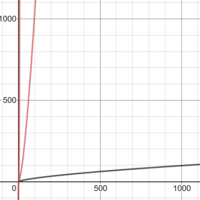That is exactly what a function and its inverse should look like! They are reflections across the line y = x; and they don't intersect except at the origin. Anything else would not represent these functions!

#### Kylian187

##### New member
You still haven't said what x and y mean!

It appears that you are making two graphs where x in the first represents $$\displaystyle \tau$$ in the formula, and y represents I, so that the equation graphed is $$\displaystyle y = 16\sqrt{I}$$; whereas in the second (inverse) plot x represents I and y represents $$\displaystyle \tau$$, so the equation is $$\displaystyle y = \frac{x^2}{256}$$.

Do you understand that your x and y do not have the same meaning in the two plots, so that they really don't make sense being on the same pair of axes?

Yes, you can scale the graphs as Mr. Bland suggests to make the graphs look the way you expect; but why do you want that? What are you going to do with a graph in which the axes have different meanings for different curves? Plotting a function and its inverse on the same axes makes sense only when the variables are abstract, treated as mere numbers, inputs and outputs.

Now, treating it abstractly, I see that the graph of your actual function and its inverse are

View attachment 16133

That is exactly what a function and its inverse should look like! They are reflections across the line y = x; and they don't intersect except at the origin. Anything else would not represent these functions!
Yes that's right and those are indeed the plots that I get as well. But what my question is this possible: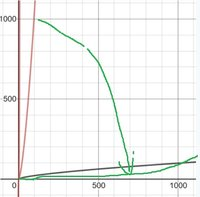So they are both aligned on the same axis. This is needed for a computer program that I am writing for percieved perception on sound. Is there a formula for this (green) line. Because for what I understood that it was the inverse but perhaps there is an other methode for achieving this.

And Dr.Peterson I really appriciate your help, sorry for my faults I am not a mathematician.

Thanks anyways both#### Dr.Peterson

##### Elite Member
Again, you need to explain why you think the green line is what you should be showing. If it is just to make both graphs visible at the same time, then some sort of scaling might be suitable; but why?

How do you want the reader to understand the meaning of that green line? What does it mean to you that both curves pass through the point (1023, 100)?

I suspect that the problem here may be that you are trying to use mathematical terms, such as "inverse", rather than just stating in everyday language what your goal is. Don't try to be a mathematician; just tell us what you want to show.

#### Kylian187

##### New member
Alright I will try my best.

Cite from: https://en.wikipedia.org/wiki/Stevens's_power_law
"Stevens's power law is an empirical relationship in psychophysics between an increased intensity or strength in a physical stimulus and the perceived magnitude increase in the sensation created by the stimulus."

What the idea is about Steven's power law is that what we see, hear, feel is not the same as the intensity that the object (such as LED or Sound) produce. For sound there is the curve is seen in the photo.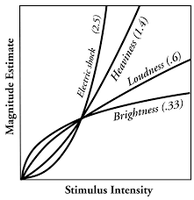So how higher the Stimulus Intensity (I) how less it makes a diffrence in what we hear. So when I is 5 and after a while 10 there is a bigger noticable diffrence than when you would use 500 and 505. Even though the diffrence in Stimulus intensity is of the same size.

For programming we would like people to hear the line X = Y. So when we step up the intensity by 1 we want the person who listens to feel the same intensity diffrence by 1. And when we would get to 500 that there is a noticable diffrence with 501.

So the theory is when the perception of hearing is calculated with Stevens power law (this is the curve how people hear) and we would invert (if that is the right term) so if you would face both curves to each (same idea as anti-sound see picutre) other people would hear X=Y .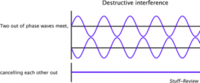So if we can program our items (Microcontroller, potmeter) to send out the inverted curve of what people hear it would end up in X = Y. So what the green line is supposed to be is the curve that will rectify other curve.

The reason why I got the number 1023 and 100 is because the Microcontroller uses 1023 (10 bits) for converting the user input (potmeter) into a percieved sound. The 100 is because there is a scale from 0% to 100% where 0% is quit/ muted, and 100% is max sound.

This is why I tried to invert the sound on a scale with 100 and 1023, and when I inverted the formula the graph was indeed inverted as I wanted but on the wrong axis because if you would add these lines thogether you won't get X=Y for the listener.

This is my problem I hope this explains everything if not let me know I will try to explain more or better.

Thanks for your help tho#### Dr.Peterson

##### Elite Member
Okay. It looks like you were right to want the inverse, and you obtained it correctly; but you were wrong to think the graphs of the function and its inverse should look like the one with four curves that you show above. That graph appears to show the same function with different values of the exponent $$\displaystyle \alpha$$. It is not intended to show a function like yours and its inverse.

You have found the inverse function you want. If you input a desired value for $$\displaystyle \tau$$ (the perceived intensity?), then your calculated intensity $$\displaystyle I_\delta(\tau)$$ will, in theory, result in the desired $$\displaystyle \tau$$ in the hearer; plug this formula in place of $$\displaystyle I$$ in the original formula and it will simplify down to $$\displaystyle I$$. In particular, if you calculate $$\displaystyle I_\delta(100)$$, you will find it is equal to 1023. That's what the graph shows, and that's what you want.

I don't think your comparison to "anti-sound" cancellation is appropriate, though. You aren't adding two signals together; rather, you are composing them, passing an input through a filter so that the output from your device will have the desired effect in the listener (whose ear or brain is performing the transformation you have inverted).

#### Kylian187

##### New member
I am not sure what you suggestWhat I thought you ment that if I would put my inverse into the original I (see picture)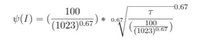When I plot this formula in my calculater it won't show the right graph. And when I put in 100 the answer was 100 instead of 1023.

Thanks for the efford of helping me

#### Dr.Peterson

##### Elite Member
When I plot this formula in my calculator it won't show the right graph. And when I put in 100 the answer was 100 instead of 1023.
If you simplify that composite formula (which is $$\displaystyle \psi(\psi^{-1}(\tau))$$), it reduces to $$\displaystyle \tau$$ -- that is, the identity function. Its graph will be what you think of as y = x. That is what it means to be an inverse: If you take any input value and run it through the inverse function, then run the result through the original function, you get back what you started with. That's what your goal was.

Here is your function that represents what the ear does to an incoming sound with intensity I: $$\displaystyle \psi(I) = \frac{100}{1023^{0.67}}\cdot I^{0.67}$$.

What you want is to take a desired perceived loudness $$\displaystyle \tau$$, and find the actual intensity $$\displaystyle I$$ that you need to produce, in order to result in that perceived loudness.

You have derived an inverse function $$\displaystyle \psi^{-1}(\tau)$$, which you have (appropriately) called $$\displaystyle I_\delta(\tau) = \sqrt[0.67]{\frac{\tau}{\frac{100}{1023^{0.67}}}} = 1023\left(\frac{\tau}{100}\right)^{1.5}$$. (Here I just simplified it; that's not important to the main point.)

What you have found is that if you take $$\displaystyle \tau = 100$$, your inverse function produces an intensity $$\displaystyle I = 1023$$, which tells you to produce your maximum intensity. When that intensity is put into the power law representing the hearer's response, it yields 100 -- the maximum loudness you want to evoke.

That's exactly what you want, right? You want the composite function $$\displaystyle \psi(\psi^{-1}(\tau))$$ to equal $$\displaystyle \tau$$, the desired loudness.

#### Kylian187

##### New member
What you have found is that if you take τ=100τ=100\displaystyle \tau = 100, your inverse function produces an intensity I=1023I=1023\displaystyle I = 1023, which tells you to produce your maximum intensity.
Yea indeed but what I need is when I read 1023 it should produce 100. If you get what I mean with it. And I am not sure how I can manage

0, 1, 3, 5, 8, 11, 15, 19, 23, 28, 32, 37, 43, 48, 54, 59,
65, 72, 78, 85, 91, 98, 106, 113, 120, 128, 136, 144, 152, 160, 168, 177,
185, 194, 203, 212, 221, 230, 240, 249, 259, 269, 278, 288, 299, 309, 319, 330,
340, 351, 362, 373, 384, 395, 406, 417, 429, 440, 452, 464, 475, 487, 499, 512,
524, 536, 549, 561, 574, 586, 599, 612, 625, 638, 651, 664, 678, 691, 705, 718,
732, 746, 760, 774, 788, 802, 816, 830, 844, 859, 873, 888, 903, 917, 932, 947,
962, 977, 992, 1008, 1023

These are 100 entries with maximum of 1023 and I need 1023 entries with maximum of 100. with the oppisite (inversed) curve of that original formula.

I tried serveral things but I can't figure it out

#### Dr.Peterson

##### Elite Member
Yea indeed but what I need is when I read 1023 it should produce 100. If you get what I mean with it. And I am not sure how I can manage

0, 1, 3, 5, 8, 11, 15, 19, 23, 28, 32, 37, 43, 48, 54, 59,
65, 72, 78, 85, 91, 98, 106, 113, 120, 128, 136, 144, 152, 160, 168, 177,
185, 194, 203, 212, 221, 230, 240, 249, 259, 269, 278, 288, 299, 309, 319, 330,
340, 351, 362, 373, 384, 395, 406, 417, 429, 440, 452, 464, 475, 487, 499, 512,
524, 536, 549, 561, 574, 586, 599, 612, 625, 638, 651, 664, 678, 691, 705, 718,
732, 746, 760, 774, 788, 802, 816, 830, 844, 859, 873, 888, 903, 917, 932, 947,
962, 977, 992, 1008, 1023

These are 100 entries with maximum of 1023 and I need 1023 entries with maximum of 100. with the opposite (inverse) curve of that original formula.

I tried several things but I can't figure it out
I don't understand what you are not understanding.

Your original function, $$\displaystyle \psi(I) = \frac{100}{1023^{0.67}}\cdot I^{0.67}$$, takes inputs from 0 to 1023 you list here (after rounding), and produces outputs 0 through 100. If you put all integers from 0 to 1023 into that function, it will produce a list of 1024 numbers from 0 to 100, which seems to be what you are asking for here. So the original function you gave fulfills your request, "when I read 1023 it should produce 100".

But here is the start of a list of 1024 numbers (values of tau, maximum 100) whose outputs (values of I) will be 0, 1, 2, ..., 1023:

I tau
0 0
1 0.96246141
2 1.531346332
3 2.009345257
4 2.436483752
5 2.829392698
6 3.197014921
7 3.544859382
8 3.876623434
9 4.194940514
10 4.501770236
... ...

That would seem to fulfill your request, "I need 1023 entries with maximum of 100". I merely put 0, 1, 2, ... into the original function.

Your inverse function, which I simplified to $$\displaystyle I(\tau) = 1023\left(\frac{\tau}{100}\right)^{1.5}$$, takes the inputs you listed, 0, 1, 3, 5, 8, ..., 1023, and produces integer outputs from 1 to 100 (after rounding). This is what I think you really want, the correct inverse function.

I put both into a spreadsheet and confirmed that both functions produce the results I just described.

What more is there to do? You've had both formulas from the beginning!

#### Kylian187

##### New member
ψ(I)=10010230.67⋅I0.67
This formula is the curve that people hear. So what I need is a list that look something like this:

0, 0, 0, 0, 0, 1, 1, 1, 1, 1, 1, 2, 2, 2, 3, 3, 3,.... etc.

with 1023 entries and a max value of 100.

Because the potmeter has a range from 0 till 1023. In the range 0 to 10 (reading potmeter). The output on the display should be 0 (because we can't hear it yet). Than the range between 10 and 17 (reading potmeter) the volume output is 1. etc etc.

What the original formula generates is the curve of what people hear but I have to counter this. And the inverted formula generates if the volume is 100 the output of the potmeter should be 1023. Instead of if the potmeter is 1023 the output volume is 100.

So the generated potmeter value should generate a certain volume display

Hope this explains what I mean?

#### Dr.Peterson

##### Elite Member
I don't think you mentioned a display until now; and the initial question said nothing about a list. Maybe this will help me figure out what you want.

I think that the list I started for you last time could be what you are describing here, except that you want it rounded. But as I said, this is just a matter of plugging numbers from 0 through 1023 into the original formula (and then rounding). Here is part of that list, with rounding:

I tau rounded
0 0 0
1 0.96246141 1
2 1.531346332 2
3 2.009345257 2
4 2.436483752 2
5 2.829392698 3
6 3.197014921 3
7 3.544859382 4
8 3.876623434 4
9 4.194940514 4
10 4.501770236 5
...
1010 99.14678724 99
1011 99.21254714 99
1012 99.27828558 99
1013 99.34400259 99
1014 99.40969819 99
1015 99.47537241 99
1016 99.54102529 100
1017 99.60665685 100
1018 99.67226711 100
1019 99.73785611 100
1020 99.80342387 100
1021 99.86897042 100
1022 99.93449579 100
1023 100 100

But this still doesn't match what I expect you to want. So I'd better describe my current understanding of what you need, so you can correct it.

As I envision your description, there is a dial the user turns, and he would expect a certain angular movement to result in a certain increase in perceived volume, so that the effect is linear. I assume your "potmeter" is what I know as a potentiometer, which produces a signal (resistance, or voltage) that increases linearly; and it is sensed in such a way that as it is turned from position 0 to a full turn, the value increases linearly from 0 to 1023. But it is labeled from 0 to 100% for the user to see.

So you want an input from there to be a signal from 0 to 1023 that represents a perceived volume from 1 to 100%, increasing linearly. Therefore, your $$\displaystyle \tau$$ is actually x*100/1023, where x is the input signal and $$\displaystyle \tau$$ is the desired percentage loudness. That's what hasn't been mentioned until now.

Then you want your system to produce a sound with intensity $$\displaystyle I$$ such that the resulting $$\displaystyle \tau = \frac{100}{1023^{0.67}}\cdot I^{0.67}$$ will be the value we just calculated from the input. That is, we want to apply the inverse function $$\displaystyle I(\tau) = 1023\left(\frac{\tau}{100}\right)^{1.5}$$ to $$\displaystyle \tau = (100/1023)x$$, to determine the intensity.

Now, what input does your system want to specify the intensity? I'd guess from what you've said that it should be an integer from 0 to 100 (though it would make more sense to me to use either a real number or something scaled larger (0 to 1000, say) to allow finer adjustment rather that going by 1% steps).

If I'm right, and the intensity should be a percentage, then you want $$\displaystyle I(x) = 100\left(\frac{(100/1023)x}{100}\right)^{1.5} = 100\left(\frac{x}{1023}\right)^{1.5}$$. Here is the table for that:

x I rounded
0 0 0
1 0.003056234 0
2 0.008644334 0
3 0.015880656 0
4 0.024449869 0
5 0.034169731 0
6 0.044917277 0
7 0.056602239 0
8 0.069154673 0
9 0.082518308 0
10 0.096646593 0
...
1010 98.09991026 98
1011 98.24563926 98
1012 98.39144034 98
1013 98.53731347 99
1014 98.68325863 99
1015 98.82927577 99
1016 98.97536485 99
1017 99.12152585 99
1018 99.26775872 99
1019 99.41406343 99
1020 99.56043995 100
1021 99.70688825 100
1022 99.85340827 100
1023 100 100

Am I getting close? I think it's still wrong because I didn't take fully into account the proper range for every variable yet.

•Kylian187

#### Kylian187

##### New member
100(x1023)1.5
This is indeed what I was looking for!!

I didn't indeed mention about the dispay because I thought it would only make it more complicated and thought it was an "easy" fix that I just didn't see.

But to give the full perspect of what for it is. This is for a build amplifier and it uses a microcontroller (PIC) with a potentiometer, display and rotary encoder. The potentiometer is the volume input of the which is translated by the microcontroller to 1023 bits. Those bits needs to be converted back into a value that the "user" can read an understand like 0 to 100% volume power. But we need to take in consideration that what people hear is not a linear line. So we use Stevens power law to calculate and the curve and need to "rectify" this curve. And the formula I qouted is indeed the formula I was looking for!

I hope I didn't bother you to much and thanks for helping!

#### Dr.Peterson

##### Elite Member
You'll want to learn how to write the specifications clearly when you ask someone else to help with a project. That is an important part of engineering (even when you're doing the design entirely by yourself, because you have to know what you mean!). That includes stating the overall context, and defining the required inputs, processing, and outputs.

In this case, the input is a number x from 0 to 1023 representing a scaled value for perceived volume (0 to 100%); the output is a number y from 0 to 100 representing the actual intensity as a percentage; and the processing is defined by your equation (restated here in terms of my x and y),

$$\displaystyle x = \frac{100}{1023^{0.67}} y^{0.67}$$​

Let's check this to make sure it fits my definitions, since I said last time that I hadn't checked that. It has the correct power-law form, so we can just check the endpoints to see that the parameters are correct. For x = 0, we want y = 0, and in fact

$$\displaystyle x = \frac{100}{1023^{0.67}} 0^{0.67} = 0$$​

For x = 1023, we want y = 100:

$$\displaystyle x = \frac{100}{1023^{0.67}} 100^{0.67} = 21$$​

Oops, not right. Your original equation didn't follow my definitions. (I didn't think so.) Let's find what the parameters should be; we want to find k in $$\displaystyle x = k\cdot y^{0.67}$$ so that $$\displaystyle 1023 = k\cdot 100^{0.67}$$. (I'm trusting that you made the right choice of a = 0.67.) Solving this, we find that the correct equation is

$$\displaystyle x = \frac{1023}{100^{0.67}} y^{0.67}$$​

which can be rewritten as

$$\displaystyle x = 1023 \left(\frac{y}{100}\right)^{0.67}$$​

Therefore, the processing needed is the inverse of this, which is what I said before (having corrected the inverse without dealing with the original):

$$\displaystyle y = 100 \left(\frac{x}{1023}\right)^{1.5}$$​

Now, at last, I can be confident of my answer, because I had something definite to check it against.